# PSEB 12th Class Physics Solutions Chapter 5 Magnetism and Matter

Punjab State Board PSEB 12th Class Physics Book Solutions Chapter 5 Magnetism and Matter Textbook Exercise Questions and Answers.

## PSEB Solutions for Class 12 Physics Chapter 5 Magnetism and Matter

PSEB 12th Class Physics Guide Magnetism and Matter Textbook Questions and Answers

Question 1.
Answer the following questions regarding earth’s magnetism:
(a) A vector needs three quantities for its specification. Name the three independent quantities conventionally used to specify the earth’s magnetic field.

(b) The angle of dip at a location in southern India is about 18°
Would you expect a greater or smaller dip angle in Britain?

(c) If you made a map of magnetic field lines at Melbourne in Australia, would the lines seem to go into the ground or come out of the ground?

(d) In which direction would a compass free to move in the vertical plane point to, if located right on the geomagnetic north or south pole?

(e) The earth’s field, it is claimed, roughly approximates the field due to a dipole of magnetic moment 8 × 1022 JT-1 located at its centre. Check the order of magnitude of this number in some way.

(f) Geologists claim that besides the main magnetic N-S poles, there are several local poles on the earth’s surface oriented in different directions. How is such a thing possible at all?
(a) The three independent quantities conventionally used for specifying earth’s magnetic field are magnetic declination, angle of dip and horizontal component of earth’s magnetic field.

(b) The angle of dip at a point depends on how far the point is located with respect to the North Pole or the South Pole. The angle of dip would be greater in Britain (it is about 70°) than in southern India because the location of Britain on the globe is closer to the magnetic North Pole.

(c) It is hypothetically considered that a huge bar magnet is dipped inside earth with its North Pole near the geographic South Pole and its South Pole near the geographic North Pole.

Magnetic field lines emanate from a magnetic North Pole and terminate at a magnetic South Pole. Hence, in a map depicting earth’s magnetic field lines, the field lines at Melbourne, Australia would seem to come out of the ground.

(d) If a compass is located on the geomagnetic North Pole or South Pole, then the compass will be free to move in the horizontal plane while earth’s field is exactly vertical to the magnetic poles. In such a case, the compass can point in any direction.

(e) Magnetic moment, M = 8 × 1022 JT-1
Radius of earth, r = 6.4 × 106 m
Magnetic field strength, B = $$\frac{\mu_{0} M}{4 \pi r^{3}}$$
∴ B = $$\frac{4 \pi \times 10^{-7} \times 8 \times 10^{22}}{4 \pi \times\left(6.4 \times 10^{6}\right)^{3}}$$ = 0.3G
This quantity is of the order of magnitude of the observed field on earth.

(f) Yes, there are several local poles on earth’s surface oriented in different directions. A magnetised mineral deposit is an example of a local N-S pole.Question 2.
(a) The earth’s magnetic field varies from point to point in space. Does it also change with time? If so, on what time scale does it change appreciably?

(b) The earth’s core is known to contain iron. Yet geologists do not regard this as a source of the earth’s magnetism. Why?

(c) The charged currents in the outer conducting regions of the earth’s core are thought to be responsible for earth’s magnetism. What might be the ’battery’ (i.e., the source of energy) to sustain these currents?

(d) The earth may have even reversed the direction of its field several times during its history of 4 to 5 billion years. How can geologists know about the earth’s field in such distant past?

(e) The earth’s field departs from its dipole shape substantially at large distances (greater than about 30,000 km). What agencies may be responsible for this distortion?

(f) Interstellar space has an extremely weak magnetic field of the order of 10-12 T. Can such a weak field he of any significant consequence? Explain.
[Note: Exercise 5.2 is meant mainly to arouse your curiosity. Answers to some questions above are tentative or unknown. Brief answers wherever possible are given at the end. For details, you should consult a good text on geomagnetism.]
(a) Earth’s magnetic field changes with time. It takes a few hundred years to change by an appreciable amount. The variation in earth’s magnetic field with the time cannot be neglected.

(b) Earth’s core contains molten iron. This form of iron is not ferromagnetic. Hence, this is not considered as a source of earth’s magnetism.

(c) The radioactivity in earth’s interior is the source of energy that sustains the currents in the outer conducting regions of earth’s core. These charged currents are considered to be responsible for earth’s magnetism.

(d) Earth reversed the direction of its field several times during its history of 4 to 5 billion years. These magnetic fields got weakly recorded in rocks during their solidification. One can get clues about the geomagnetic history from the analysis of this rock magnetism.

(e) Earth’s field departs from its dipole shape substantially at large distances (greater than about 30,000 km) because of the presence of the ionosphere. In this region, earth’s field gets modified because of the field of single ions. While in motion, these ions produce the magnetic field associated with them.

(f) An extremely weak magnetic field can bend charged particles moving in a circle. This may not be noticeable for a large radius path. With reference to the gigantic interstellar space, the deflection can affect the passage of charged particles.

Question 3.
A short bar magnet placed with its axis at 30° with a uniform external magnetic field of 0.25 T experiences a torque of magnitude equal to 4.5 × 10-2 J. What is the magnitude of magnetic moment of the magnet?
Magnetic field strength, B = 0.25 T
Torque on the bar magnet, τ = 4.5 × 10-2J
Angle between the bar magnet and the external magnetic field, θ = 30°
Torque is related to magnetic moment (M) as
τ = MB sinθ
∴ M = $$\frac{\tau}{B \sin \theta}$$
= $$\frac{4.5 \times 10^{-2}}{0.25 \times \sin 30^{\circ}}$$
$$\frac{4.5 \times 10^{-2} \times 2}{0.25 \times 1}$$
(∵ sin30° = $$\frac{1}{2}$$)
= 0.36 JT-1
Hence, the magnetic moment of the magnet is 0.36 JT-1.Question 4.
A short bar magnet of magnetic moment m = 0.32 JT-1 is placed in a uniform magnetic field of 0.15 T. If the bar is free to rotate in the plane of the field, which orientation would correspond to its (a) stable, and (b) unstable equilibrium? What is the potential energy of the magnet in each case?
Given, M = 0.32 JT-1, B = 0.15T,U = ?
(a) Stable Equilibrium: The magnetic moment should be parallel to the magnetic field. In this position, the potential energy is
U = -MB cos θ =0.32 × 0.15 × 1
= -0.048 J or-4.8 × 10-2 J\

(b) Unstable Equilibrium: The magnetic moment should be antiparallel to the magnetic field. In this position, the potential energy is
U = -MBcosθ = 0.32 × 0.15 × (-1)
= +0.048 J or + 4.8 × 10-2 J

Question 5.
A closely wound solenoid of 800 turns and area of cross-section 2.5 × 10-4 m2 carries a current of 3.0 A. Explain the sense in which the solenoid acts like a bar magnet. What is its associated magnetic moment?
Solenoid acts as a bar magnet, its magnetic moment is along the axis of the solenoid, the direction determined by the sense of flow of current. The magnetic moment of a current carrying loop having N turns
= NIA = 800 × 3 × 2.5 × 10-4
= 6 × 10-1
= 0.60 A-m2
= 0.60 JT-1

Question 6.
If the solenoid in Exercise 5.5 is free to turn about the vertical direction and a uniform horizontal magnetic field of 0.25 T is applied, what is the magnitude of torque on the solenoid when its axis makes an angle of 30° with the direction of applied field?
Magnetic field strength, B = 0.25 T
Magnetic moment, M = 0.6 JT-1
The angle θ, between the axis of the solenoid and the direction of the applied field is 30°.
Therefore, the torque acting on the solenoid is given as
τ = MB sinθ
= 0.6 × 0.25 × sin30°
= 0.6 × 0.25 × $$\frac{1}{2}$$
= 0.075 N-mQuestion 7.
A bar magnet of magnetic moment 1.5 J T-1 lies aligned with the direction of a uniform magnetic field of 0.22 T.
(a) What is the amount of work required by an external torque to turn the magnet so as to align its magnetic moment:
(i) normal to the field direction,
(ii) opposite to the field direction?

(b) What is the torque on the magnet in cases (i) and (ii)?
Given, M = 1.5 JT-1,B = 0.22 T,θ1 =0°

(a) To align the dipole normal to the field direction θ2 = 90°. Therefore,
W = MB(cosθ1 – cosθ2)
W = 1.5 × 0.22(cos0° – cos90°) = 0.33 J
Also, τ = MB sinθ2
or τ = 1.5 × 0.22sin90° = 0.33 Nm

(b) To align the dipole opposite to the field direction θ2 = 180°. Therefore,
W =MB(cosθ1 – cosθ2)
W = 1.5 × 0.22(cos0° – cos180°) = 0.66 J
Also, τ = MB sinθ2
or τ = 1.5 × 0.22sinl80° = 0 Nm

Question 8.
A closely wound solenoid of2000 turns and area of cross-section 1.6 × 10-4 m2, carrying a current of 4.0 A, is suspended through its centre allowing it to turn in a horizontal plane.
(a) What is the magnetic moment associated with the solenoid?
(b) What is the force and torque on the solenoid if a uniform horizontal magnetic field of 7.5 × 10-2 T is set up at an angle of 30° with the axis of the solenoid?
Number of turns on the solenoid, N = 2000
Area of cross-section of the solenoid, A = 1.6 × 10-4 m2</sup
Current in the solenoid, I = 4 A

(a) Let M = magnetic moment of the solenoid.
∴ Using the relation M = NIA, we get
M = 2000 × 4.0 × 1.6 × 10-4</sup
= 1.28 JT-1</sup

The direction of $$\vec{M}$$ is along the axis of the solenoid in the direction related to the sense of current according to right-handed screw rule.

(b) Here θ = 30°
$$\vec{B}$$ = 7.5 × 10-2 T
Let F = force on the solenoid = ?
τ = torque on the solenoid = ?
The solenoid behaves as a bar magnet placed in a uniform magnetic field, so the force is
F = m $$\vec{B}$$ + (-m $$\vec{B}$$) = 0
where m = pole strength of the magnet.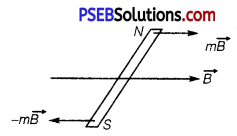Using the relation, τ = MB sinθ, we get
τ = 1.28 × 7.5 × 10-2 × sin30°
= 1.28 × 7.5 × 10-2 × $$\frac{1}{2}$$ = 0.048 J
The direction of the torque is such that it tends to align the axis of the solenoid (i. e., magnetic moment vector $$\vec{M}$$) along $$\vec{B}$$.Question 9.
A circular coil of 16 turns and radius 10 cm carrying a current of 0.75 A rests with its plane normal to an external field of magnitude 5.0 × 10-2 T. The coil is free to turn about an axis in its plane perpendicular to the field direction. When the coil is turned slightly and released, it oscillates about its stable equilibrium with a frequency of 2.0 s-1. What is the moment of inertia of the coil about its axis of rotation?
Number of turns in the circular coil, N = 16
Radius of the coil, r = 10cm = 0.1m
Cross-section of the coil, A = πr2 = π × (0.1)2 m2
Current in the coil, I = 0.75 A
Magnetic field strength, B = 5.0 × 10-2 T
Frequency of oscillations of the coil, v = 2.0 s-1
∴ Magnetic moment, M = NIA = 16 × 0.75 × π × (0.1)2 = 0.377 JT-1
Frequency is given by the relation
v = $$\frac{1}{2 \pi} \sqrt{\frac{M B}{I}}$$
where, I = Moment of inertia of the coil
I = $$\frac{M B}{4 \pi^{2} v^{2}}$$ = $$\frac{0.377 \times 5 \times 10^{-2}}{4 \pi^{2} \times(2)^{2}}$$
= 1.19 × 10-4 kg m2
Hence, the moment of inertia of the coil about its axis of rotation is 1.19 × 10-4 kg m2.

Question 10.
A magnetic needle free to rotate in a vertical plane parallel to the magnetic meridian has its north tip pointing down at 22° with the horizontal. The horizontal component of the earth’s magnetic field at the place is known to be 0.35 G. Determine the magnitude of the earth’s magnetic field at the place.
Horizontal component of earth’s magnetic field, BH = 0.35 G
Angle made by the needle with the horizontal plane
= Angle of dip = δ = 22°
Earth’s magnetic field strength = B
We can relate B and BH as
BH = B cosδ
∴ B = $$\frac{B_{H}}{\cos \delta}=\frac{0.35}{\cos 22^{\circ}}=\frac{0.35}{0.9272}$$ = 0.377 G
Hence, the strength of earth’s magnetic field at the given location is 0.377 G.

Question 11.
At a certain location in Africa, a compass points 12° west of the geographic north. The north tip of the magnetic needle of a dip circle placed in the plane of magnetic meridian points 60° above the horizontal. The horizontal component of the earth’s field is measured to he 0.16 G. Specify the direction and magnitude of the earth’s field at the location.
Angle of declination, θ = 12°
Angle of dip, δ = 60°
Horizontal component of earth’s magnetic field, BH = 0.16 G
Earth’s magnetic field at the given location = B
We can relate B and BH as
BH = B cosδ
B= $$\frac{B_{H}}{\cos \delta}$$ = $$=\frac{0.16}{\cos 60^{\circ}}$$ = $$\frac{0.16}{\left(\frac{1}{2}\right)}$$ = 0.16 × 2 = 0.32 G
Earth’s magnetic field lies in the vertical plane, 12° west of the geographic meridian, making an angle of 60° (Upward) with the horizontal direction. Its magnitude is 0.32 G.Question 12.
A short bar magnet has a magnetic moment of 0.48 JT-1. Give the direction and magnitude of the magnetic field produced by the magnet at a distance of 10 cm from the centre of the magnet on (a) the axis, (b) the equatorial lines (normal bisector) of the magnet.
Magnetic moment of the bar magnet, M = 0.48 JT-1
Distance, d = 10cm = 0.1m

(a) The magnetic field at distance d, from the centre of the magnet on the axis is given by the relation,
B = $$\frac{\mu_{0}}{4 \pi} \frac{2 M}{d^{3}}$$
∴ B = $$\frac{4 \pi \times 10^{-7} \times 2 \times 0.48}{4 \pi \times(0.1)^{3}}$$
= 0.96 × 10-4 T = 0.96 G
The magnetic field is along the S-N direction.

(b) The magnetic field at a distance of 10 cm (i.e., d = 0.1 m) on the equatorial line of the magnet is given as,
B = $$\frac{\mu_{0} \times M}{4 \pi \times d^{3}}$$
∴ B = $$\frac{4 \pi \times 10^{-7} \times 0.48}{4 \pi \times(0.1)^{3}}$$ = 0.48G
The magnetic field is along the N-S direction.

Question 13.
A short bar magnet placed in a horizontal plane has its axis aligned along the magnetic north-south direction. Null points are found on the axis of the magnet at 14 cm from the centre of the magnet. The earth’s magnetic field at the place is 0.36 G and the angle of dip is zero. What is the total magnetic field on the normal bisector of the magnet at the same distance as the null-point (i. e., 14 cm) from the centre of the magnet? (At null points, field due to a magnet is equal and opposite to the horizontal component of earth’s magnetic field.)
Distance of the null point from the centre of magnet
d = 14 cm = 0.14 m
The earth’s magnetic field where the angle of dip is zero, is the horizontal component of earth’s magnetic field. i.e., H = 0.36 G
Initially, the null points are on the axis of the magnet. We use the formula of magnetic field on axial line (consider that the magnet is short in length).
B1 = $$\frac{\mu_{0}}{4 \pi} \cdot \frac{2 m}{d^{3}}$$
This magnetic field is equal to the horizontal component of earth’s magnetic field.
i.e., B1 = $$\frac{\mu_{0}}{4 \pi} \cdot \frac{2 m}{d^{3}}$$ = H ……….(1)
On the equitorial line of magnet at same distance (d) magnetic field due to the magnet
B2 = $$\frac{\mu_{0}}{4 \pi} \cdot \frac{m}{d^{3}}=\frac{B_{1}}{2}=\frac{H}{2}$$ …………….. (2)
The total magnetic field on equitorial line at this point (as given in question)
B = B2 + H = $$\frac{H}{2}$$ + H = $$\frac{3}{2}$$H = $$\frac{3}{2}$$ × 0.36 = 0.54G
The direction of magnetic field is in the direction of earth’s field.

Question 14.
If the bar magnet in exercise 5.13 is turned around by 180°, where will the new null points be located?
When the bar magnet is turned by 180°, then the null points are obtained on the equitorial line.
So, magnetic field on the equitorial line at distance d’ is
B’ = $$\frac{\mu_{0}}{4 \pi} \cdot \frac{m}{d^{3}}$$
This magnetic field is equal to the horizontal component of earth’s magnetic field
B’ = $$\frac{\mu_{0}}{4 \pi} \cdot \frac{m}{d^{3}}$$ = H ………… (1)
From Q.No. 13 MISS
Magnetic field B1 = $$\frac{\mu_{0}}{4 \pi} \cdot \frac{2 m}{d^{3}}$$ = H ………….. (2)
From eqs. (1) and (2), we get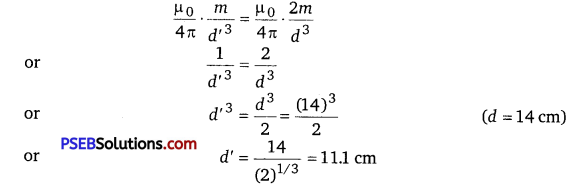Thus, the null points are located on the equitorial line at a distance of 11.1 cm.Question 15.
A short bar magnet of magnetic moment 5.25 × 10-2 JT-1 is placed with its axis perpendicular to the earth’s field direction. At what distance from the centre of the magnet, the resultant field is inclined at 45° with earth’s field on (a) its normal bisector and (b) its axis. Magnitude of the earth’s field at the place is given to be 0.42 G. Ignore the length of the magnet in comparison to the distances involved.
Given, magnetic moment m = 5.25 × 10-2 J/T
Let the resultant magnetic field is Bnet. It makes an angle of 45° with Be.
∴ Be = 0.42G =0.42 × 10-4 T
(a) At normal bisector
Let r is the distance between axial line and point P.
The magnetic field at point P, due to a short magnet
B = $$\frac{\mu_{0}}{4 \pi} \cdot \frac{m}{r^{3}}$$ …………. (1)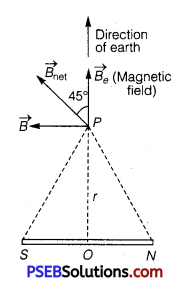The direction of B is along PB, i.e., along N pole to S pole.
According to the vector analysis,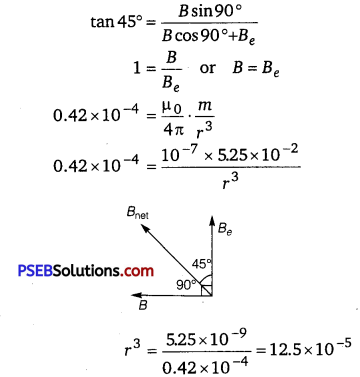r = 0.05 m
r = 5 cm

(b) When point lies on axial line
Let the resultant magnetic field Bnet makes an angle 45° from Be. The magnetic field on the axial line of the magnet at a distance of r from the centre of magnet
B’ = $$\frac{\mu_{0}}{4 \pi} \cdot \frac{2 m}{r^{3}}$$ (S to N)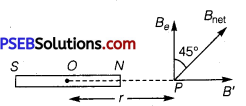Direction of magnetic field is from S to N.
According to the vector analysis,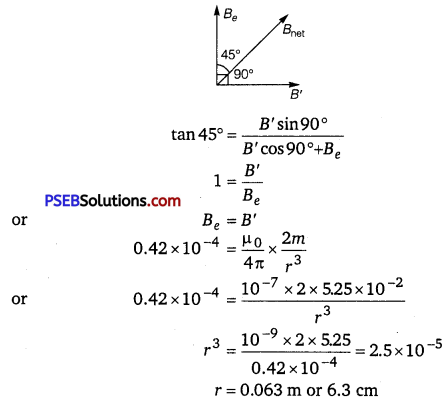Question 16.
(a) Why does a paramagnetic sample display greater magnetisation (for the same magnetising field) when cooled?

(b) Why is diamagnetism, in contrast, almost independent of temperature?

(c) If a toroid uses bismuth for its core, will the field in the core be (slightly) greater or (slightly) less than when the core is empty?

(d) Is the permeability of a ferromagnetic material independent of the magnetic field? If not, is it more for lower or higher fields?

(e) Magnetic field lines are always nearly normal to the surface of a ferromagnet at every point. (This fact is analogous to the static electric field lines being normal to the surface of a conductor at every point). Why?

(f) Would the maximum possible magnetisation of a paramagnetic sample be of the same order of magnitude as the magnetisation of a ferromagnet?
(a) Owing to the random thermal motion of molecules, the alignments of dipoles get disrupted at high temperatures. On cooling, this disruption is reduced.’Hence, a paramagnetic sample displays greater magnetisation when cooled.

(b) The induced dipole moment in a diamagnetic substance is always opposite to the magnetising field. Hence, the internal motion of the atoms (which is related to the temperature) does not affect the diamagnetism of a material.

(c) Bismuth is a diamagnetic substance. Hence, a toroid with a bismuth core has a magnetic field slightly greater than a toroid whose core is empty.

(d) The permeability of ferromagnetic materials is not independent of the applied magnetic field. It is greater for a lower field and vice versa.

(e) The permeability of ferromagnetic materials is not less than one. It is always greater than one. Hence, magnetic field lines are always nearly normal to the surface of such materials at every point.

(f) The maximum possible magnetisation of a paramagnetic sample can be of the same order of magnitude as the magnetisation of a ferromagnet. This requires high magnetising fields for saturation.

Question 17.
(a) Explain qualitatively on the basis of domain picture the irreversibility in the magnetisation curve of a ferromagnet.

(b) The hysteresis loop of a soft iron piece has a much smaller area than that of a carbon steel piece. If the material is to go through repeated cycles of magnetisation, which piece will dissipate greater heat energy?

(c) ‘A system displaying a hysteresis loop such as a ferromagnet, is a device for storing memory?’ Explain the meaning of this statement.

(d) What kind of ferromagnetic material is used for coating magnetic tapes in a cassette player,’ or for building ‘memory stores’ in a modern computer?

(e) A certain region of space is to be shielded from magnetic fields. Suggest a method.
(a) The hysteresis curve (B-H curve) of a ferromagnetic material is shown in the following figure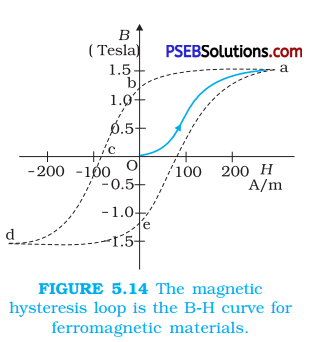It can be observed from the given curve that magnetisation persists even when the external field is removed. This reflects the irreversibility of a ferromagnet.

(b) The dissipated heat energy is directly proportional to the area of a hysteresis loop. A carbon steel piede has a greater hysteresis curve area. Hence, it dissipates greater heat energy.

(c) The value of magnetisation is memory or record of hysteresis loop cycles of magnetisation. These bits of information correspond to the cycle of magnetisation. Hysteresis loops can be used for storing information.

(d) Ceramic is used for coating magnetic tapes in cassette players and for building memory stores in modern computers.

(e) A certain region of space can be shielded from magnetic fields if it is – surrounded by soft iron rings. In such arrangements, the magnetic lines are drawn out of the region.Question 18.
A long straight horizontal cable carries a current of 2.5 A in the direction 10° south of west to 10° north of east. The magnetic meridian of the place happens to be 10° west of the geographic meridian. The earth’s magnetic field at the location is 0.33 G, and the angle of dip is zero. Locate the line of neutral points (ignore the thickness of the cable). (At neutral points, magnetic field due to a current-carrying cable is equal and opposite to the horizontal component of earth’s magnetic field.)
Given, current in the cable
I = 2.5 A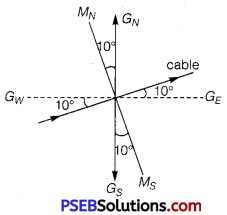Magnetic meridian MNMS is 10° west of geographical meridian GNGS earth’s magnetic field R = 0.33 G
= 0.33 × 10-4 T ……….. (1)
Angle of dip δ = S
The neutral point is the point where the magnetic field due to the current carrying cable is equal to the horizontal component of earth’s magnetic field.
Horizontal component of earth’s magnetic field
H = Rcosθ = 0.33 × 10-4 cos0°
= 0.33 × 10-4 T
Using the formula of magnetic field at distance r due to an infinite long current carrying conductor
B = $$\frac{\mu_{0}}{4 \pi} \cdot \frac{2 I}{r}$$
At neutral points,
H = B
0.33 × 10-4 = $$\frac{\mu_{0}}{4 \pi} \cdot \frac{2 I}{r}$$
0.33 × 10-4 = $$\frac{10^{-7} \times 2 \times 2.5}{r}$$
or r = $$\frac{5 \times 10^{-7}}{0.33 \times 10^{-4}}$$
or r = 1.5 × 10-2 m = 1.5cm
Thus, the line of neutral points is at a distance of 1.5 cm from the cable.

Question 19.
A telephone cable at a place has four long straight horizontal wires carrying a current of 1.0 A in the same direction east to west. The earth’s magnetic field at the place is 0.39 G, and the angle of dip is 35°. The magnetic declination is nearly zero. What are the resultant magnetic fields at points 4.0 cm above and below the cable?
Number of horizontal wires in the telephone cable, n = 4
Current in each wire, I = 1.0 A
Earth’s magnetic field at the location, H = 0.39 G = 0.39 × 10-4 T
Angle of dip at the location, δ = 35°
Angle of declination, θ = 0°
For a point 4 cm below the cable
Distance, r = 4 cm = 0.04 m
The horizontal component of earth’s magnetic field can be written as
Hh, = Hcosδ – B
where,
B = Magnetic field at 4 cm due to current I in the four wires
= 4 × $$\frac{\mu_{0} I}{2 \pi r}$$
μ0 = 4π × 10-7 TmA-1
∴ B = 4 × $$\frac{4 \pi \times 10^{-7} \times 1}{2 \pi \times 0.04}$$
= 0.2 × 10-4 T = 0.2G
∴ Hh = 0.39 cos35°- 0.2
= 0.39 × 0.819 – 0.2 ≈ 0.12 G

The vertical component of earth’s magnetic field is given as
Hv = H sinδ
= 0.39 sin35°= 0.22 G
The angle made by the field with its horizontal component is given as
θ = tan-1 $$\frac{H_{v}}{H_{b}}$$
= tan-1 $$\frac{0.22}{0.12}$$ = 61.39°
The resultant field at the point is given as
H1 = $$\sqrt{\left(H_{v}\right)^{2}+\left(H_{h}\right)^{2}}$$
= $$\sqrt{(0.22)^{2}+(0.12)^{2}}$$ = 0.25 G

For a point 4 cm above the cable
Horizontal component of earth’s magnetic field
Hh = Hcosδ +B = 0.39 cos35° + 0.2 = 0.52 G
Vertical component of earth’s magnetic field
Hv = H sinδ
= 0.39 sin35° = 0.22 G
Angle, θ = tan-1 $$\frac{H_{v}}{H_{h}}$$ = tan-1$$\frac{0.22}{0.52}$$ = 22.90
And resultant field
H2 = $$\sqrt{\left(H_{v}\right)^{2}+\left(H_{h}\right)^{2}}$$
= $$\sqrt{(0.22)^{2}+(0.52)^{2}}$$ = 0.56 GQuestion 20.
A compass needle free to turn in a horizontal plane is placed at the centre of circular coil of 30 turns and radius 12 cm. The coil is in a vertical plane making an angle of 45° with the magnetic meridian. When the current in the coil is 0.35 A, the needle points west to east.
(a) Determine the horizontal component of the earth’s magnetic field at the location.
(b) The current in the coil is reversed, and the coil is rotated about its vertical axis by an angle of 90° in the anticlockwise sense looking from above. Predict the direction of the needle. Take the magnetic declination at the places to be zero.
Number of turns in the circular coil, N = 30
Radius of the circular coil, r = 12cm = 0.12m
Current in the coil, I = 0.35 A
Angle of dip, δ = 45°

(a) The magnetic field due to current I, at a distance r, is given as
B = $$\frac{\mu_{0} 2 \pi N I}{4 \pi r}$$
∴ B = $$\frac{4 \pi \times 10^{-7} \times 2 \pi \times 30 \times 0.35}{4 \pi \times 0.12}$$
= 5.49 × 10-5 T
The compass needle points from west to east. Hence, the horizontal component of earth’s magnetic field is given as
BH = B sinδ
= 5.49 × 10-5 sin 45°
= 3.88 × 10-5 T = 0.388G

(b) When the current in the coil is reversed and the coil is rotated about its vertical axis by an angle of 90°, the needle will reverse its original direction. In this case, the needle will point from east to west.

Question 21.
A magnetic dipole is under the influence of two magnetic fields. The angle between the field directions is 60°, and one of the fields has a magnitude of 1.2 × 10-2 T. If the dipole comes to stable equilibrium at an angle of 15° with this field, what is the magnitude of the other field?
The two fields $$\vec{B}$$1 and $$\vec{B}$$2 are shown in the figure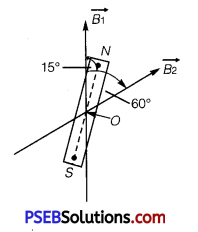here in which a magnet is placed s.t.
∠NOB = 15°
∠B1OB2 = 60°
∴ ∠NOB2 = 60 – 15 = 45°
B1 = 1.2 × 10-2 T
B2 = ?
Let θ1 and θ2 he the inclination of the dipole
with $$\vec{B}$$1 and $$\vec{B}$$2 respectively.
∴ θ1 = 15°,θ2 = 45°
If τ1 and τ2 be the torques on the dipole due to $$\vec{B}$$1 and $$\vec{B}$$2 respectively, then
Using the relation,
τ = MB sin θ, we get
τ1 = MB1 sinθ1
and τ2 = MB2 sinθ2
As the dipole is in equilibrium, the torques on the dipole due to $$\vec{B}$$1 and $$\vec{B}$$2 are equal and opposite, i. e.,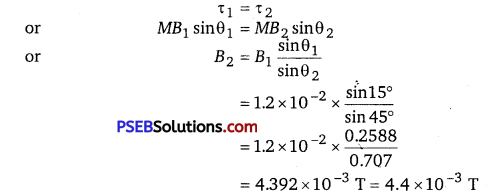Question 22.
A monoenergetic (18 keV) electron beam initially in the horizontal direction is subjected to a horizontal magnetic field of 0.40 G normal to the initial direction. Estimate the up or down deflection of the beam over a distance of 30 cm (me = 9.11 × 10-31 kg). [Note : Data in this exercise are so chosen that the answer will give you an idea of the effect of earth’s magnetic field on the motion of the electron beam from the electron gun to the screen in a TV set.]
Here, energy = E = 18 KeV = 18 × 1.6 × 10-16 J
(∵ 1 KeV=103eV = 103 × 1.6 × 10-19 J)
B = horizontal magnetic field = 0.40 G = 0.40 × 10-4 J
m = 9.11 × 10-31 kg, e = 1.6 × 10-19 C
x = 30 cm = 0.30 m
As the magnetic field is normal to the velocity, the charged particle follows circular path in magnetic field. The centrepetal force $$\frac{m v^{2}}{r}$$ required for this purpose is provided by force on electron due to magnetic field i. e., BeV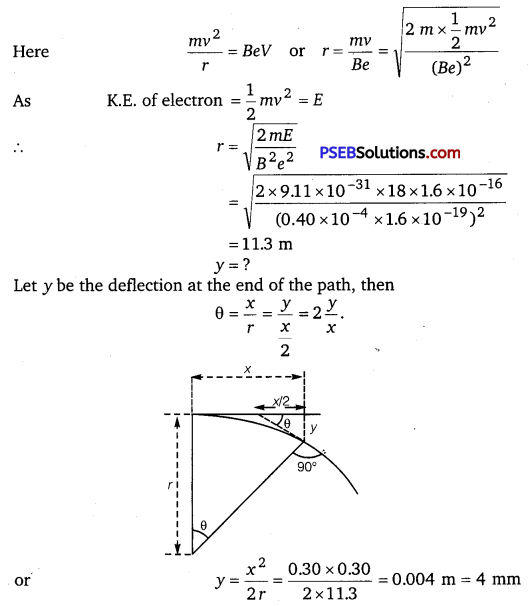Question 23.
A sample of paramagnetic salt contains 2.0 × 1024 atomic dipoles each of dipole moment 1.5 × 10-23 JT-1. The sample is placed under a homogeneous magnetic field of 0.64 T, and cooled to a temperature of 4.2 K. The degree of magnetic saturation achieved is equal to 15%. What is the total dipole moment of the sample for a magnetic field of 0.98 T and a temperature of 2.8 K? (Assume Curie’s law)
Number of atomic dipoles, n = 2.0 × 1024
Dipole moment of each atomic dipole, M = 1.5 × 10-23 JT-1
When the magnetic field, B1 = 0.64 T
The sample is cooled to a temperature, T1 = 4.2 K
Total dipole moment of the atomic dipole, Mtot = n × M
= 2 × 1024 × 1.5 × 10-23 = 30 JT-1
Magnetic saturation is achieved at 15%.
Hence, effective dipole moment, M1 = $$\frac{15}{100}$$ × 30 = 4.5 JT-1
When the magnetic field, B2 = 0.98 T
Temperature, T2 = 2.8 K
Its total dipole moment = M2
According to Curie’s law, we have the ratio of two magnetic dipoles as
$$\frac{M_{2}}{M_{1}}=\frac{B_{2}}{B_{1}} \times \frac{T_{1}}{T_{2}}$$
∴ M2 = $$\frac{B_{2} T_{1} M_{1}}{B_{1} T_{2}}$$ = $$\frac{0.98 \times 4.2 \times 4.5}{2.8 \times 0.64}$$ = 10 336 JT-1
Therefore, 10.336 J T-1 is the total dipole moment of the sample for a magnetic field of 0.98 T and a temperature of 2.8 K.

Question 24.
A Rowland ring of mean radius 15 cm has 3500 turns of wire wound on a ferromagnetic core of relative permeability 800. What is the magnetic field B in the core for a magnetising current of 1.2 A?
Mean radius of the Rowland ring, r = 15 cm = 0.15 m
Number of turns on the ferromagnetic core, N = 3500
Relative permeability of the core material, μr = 800
Magnetising current, I = 1.2 A
The magnetic field is given by the relation
B = $$\frac{\mu_{r} \mu_{0} I N}{2 \pi r}$$
B = $$\frac{800 \times 4 \pi \times 10^{-7} \times 1.2 \times 3500}{2 \pi \times 0.15}$$ = 4.48T
Therefore, the magnetic field in the core is 4.48 T.Question 25.
The magnetic moment vectors μs and μl associated with the intrinsic spin angular momentum S and orbital angular momentum l, respectively, of an electron are predicted by quantum theory (and verified experimentally to a high accuracy) to be given by
μg = -(e/m)S, μl = -(e/2m)l
Which of these relations is in accordance with the result expected classically? Outline the derivation of the classical result.
Out of these two relations, $$\overrightarrow{\mu_{l}}=-\frac{e}{2 m} \vec{l}$$ is in accordance with classical physics and can be derived as follows

We know that electrons revolving around the nucleus of an atom in circular orbits behave as tiny current loops having angular momentum $$\vec{\imath}$$ given in magnitude as
$$\vec{\imath}$$ = mvr …………. (1)
where m = mass of an electron
v = its orbital velocity
r = radius of the circular orbit.
or vr = $$\frac{l}{m}$$ …………… (2)

$$\vec{\imath}$$ acts along the normal to the plane of the orbit in upward direction. The orbital motion of electron is taken as equivalent to the flow of conventional current I given by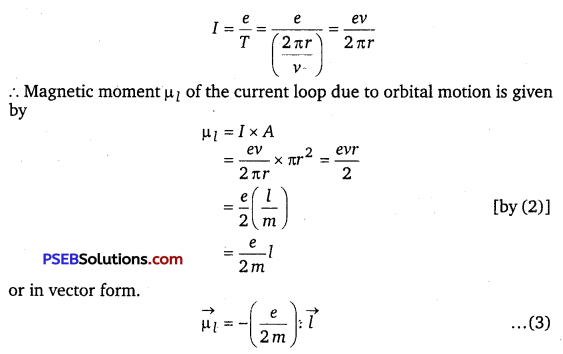where – ve sign shows that the electron is negatively
charged. The eqn. (3) shows that μe and $$\vec{\imath}$$ are opposite to each other i. e., antiparallel and both being normal to the plane of the orbit as shown in the figure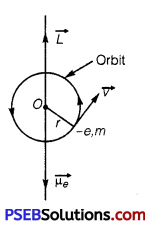∴ $$\overrightarrow{\mu_{l}}=-\frac{e}{2 m} \cdot \vec{l}$$
$$\frac{\mu_{s}}{S}$$ in contrast to $$\frac{\mu_{l}}{\vec{l}}$$ is $$\frac{e}{m}$$ i.e., twice the classically
expected value. This latter result is an outstanding consequence of modern quantum theory and cannot be obtained classically.